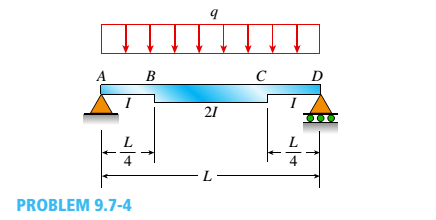# -4 A simple beam ABCD has moment of inertia I near the supports and moment of iertia 2I in the middle region, as shown in the figure. A uniform load of intensity q acts over the entire length of the beam. Determine the quations of the deflection curve for the left-hand half of the beam. Also, find the angle of rotation θ A at the left-hand support and the deflection δ max at the midpoint.### Mechanics of Materials (MindTap Co...

9th Edition
Barry J. Goodno + 1 other
Publisher: Cengage Learning
ISBN: 9781337093347### Mechanics of Materials (MindTap Co...

9th Edition
Barry J. Goodno + 1 other
Publisher: Cengage Learning
ISBN: 9781337093347
Chapter 9, Problem 9.7.4P
Textbook Problem
33 views

## -4 A simple beam ABCD has moment of inertia I near the supports and moment of iertia 2I in the middle region, as shown in the figure. A uniform load of intensity q acts over the entire length of the beam.Determine the quations of the deflection curve for the left-hand half of the beam. Also, find the angle of rotation θ A at the left-hand support and the deflection    δ max at the midpoint.

To determine

The equations of the deflection curve for the left-hand half of the beam, the angle of rotation θA at the left-hand support and the deflection δmax at the midpoint should be determined for given simple beam.

### Explanation of Solution

Given Information:

We have the simple beam ABCD with moment of inertia I near supports and moment of inertia 2I in the middle region as per below figure:

For the given simple beam below is the free body diagram.

For the above diagram, applying the force equilibrium in horizontal direction, we will get,

Fx=0Ax=0

At the point A, we are applying moment equilibrium,

MA=0;Dy×LqL×L2=0Dy=qL2

Again we are applying the force equilibrium but in vertical direction,

Fy=0AyqL+Dy=0AyqL+qL2=0Ay=qL2

Now we are taking a section which is at distance of x from point A where (0xL4) , we get below figure:

In above section, we are applying moment equilibrium,

M+qx(x2)qL2(x)=0M=qL2xq2x2EIv''=qL2xq2x2( From moment-curvature relationship)

We can get the slope equation after integrating the above equation.

EIv'=qL2x22q2x33+C1v'=1EI(qL4x2q6x3+C1)

Once again taking integration on both sides, we can determine the deflection equation,

v'=1EI(qL2 x 22q2 x 33+C1)v=1EI(qL4 x 33q6 x 44+C1x+C2)v=1EI(qL12x3q24x4+C1x+C2)

Now we are taking a section which is at distance of x from point A where (L4xL2) , we get below figure:

In above section, we are applying moment equilibrium,

M+qx(x2)qL2(x)=0M=qL2xq2x2E(2I)v''=qL2xq2x2( From moment-curvature relationship)

We can get the slope equation after integrating the above equation.

E(2I)v''=qL2xq2x2E(2I)v'=qL2x22q2x33+C3v'=12EI(qL4x2q6x3+C3)

Once again taking integration on both sides, we can determine the deflection equation,

v'=12EI(qL4x2q6x3+C3)v=12EI(qL4 x 33q6 x 44+C3x+C4)v=12EI(qL12x3q24x4+C3x+C4)

The exaggerated elastic curve for the simple beam ABCD can be represented as below diagram:

The boundary condition is applied for deflection equation for (0xL4) where deflection is zero at point A. We will get,

v=1EI(qL12x3q24x4+C1x+C2)Putting values of x =0 and v =00=1EI(qL1203q2404+C1×0+C2)C2=0

This concludes that the slope contains value as zero when the maximum deflection of the beam δmax occurs at x=L2 due to symmetry of loading.

Now applying boundary condition to deflection equation (L4xL2) where v'(L2)=0 ,

E(2I)v'=qL2x22q2x33+C3E(2I)(0)=qL4(L2)2q6(L2)3+C30=qL163qL483+C3C3=qL243

We are applying the continuity of slope condition at point B by evaluating the slope equations for segments (0xL4) and (L4xL2) as follow.

(vB')left=(vB')right1EI(qL4x2q6x3+C1)=12EI(qL4x2q6x3+C3)Putting values of x =L4and C3=qL3241EI(qL4( L 4 )2q6( L 4 )3+C1)=12EI(qL4( L 4 )2q6( L 4 )3+C3)(q L 364q L 3384+C1)=12(q L 364q L 3384q L 324)5qL3384+C1=11qL3768C1=7qL3256

Now we are applying the continuity of deflection condition at point B by evaluating the slope equations for segments (0xL4) and (L4xL2) as follow

### Still sussing out bartleby?

Check out a sample textbook solution.

See a sample solution

#### The Solution to Your Study Problems

Bartleby provides explanations to thousands of textbook problems written by our experts, many with advanced degrees!

Get Started

Find more solutions based on key concepts
Explain the differences among data, information, and a database.

Database Systems: Design, Implementation, & Management

For Problems 16.33 through 16.38, draw the isometric view of the following objects. Make the necessary measurem...

Engineering Fundamentals: An Introduction to Engineering (MindTap Course List)

What is a browser? Describe the purpose of a search engine.

Enhanced Discovering Computers 2017 (Shelly Cashman Series) (MindTap Course List)

Give one advantage of using electrodes with cellulose-based fluxes.

Welding: Principles and Applications (MindTap Course List)

If your motherboard supports ECC DDR3 memory, can you substitute non-ECC DDR3 memory?

A+ Guide to Hardware (Standalone Book) (MindTap Course List)